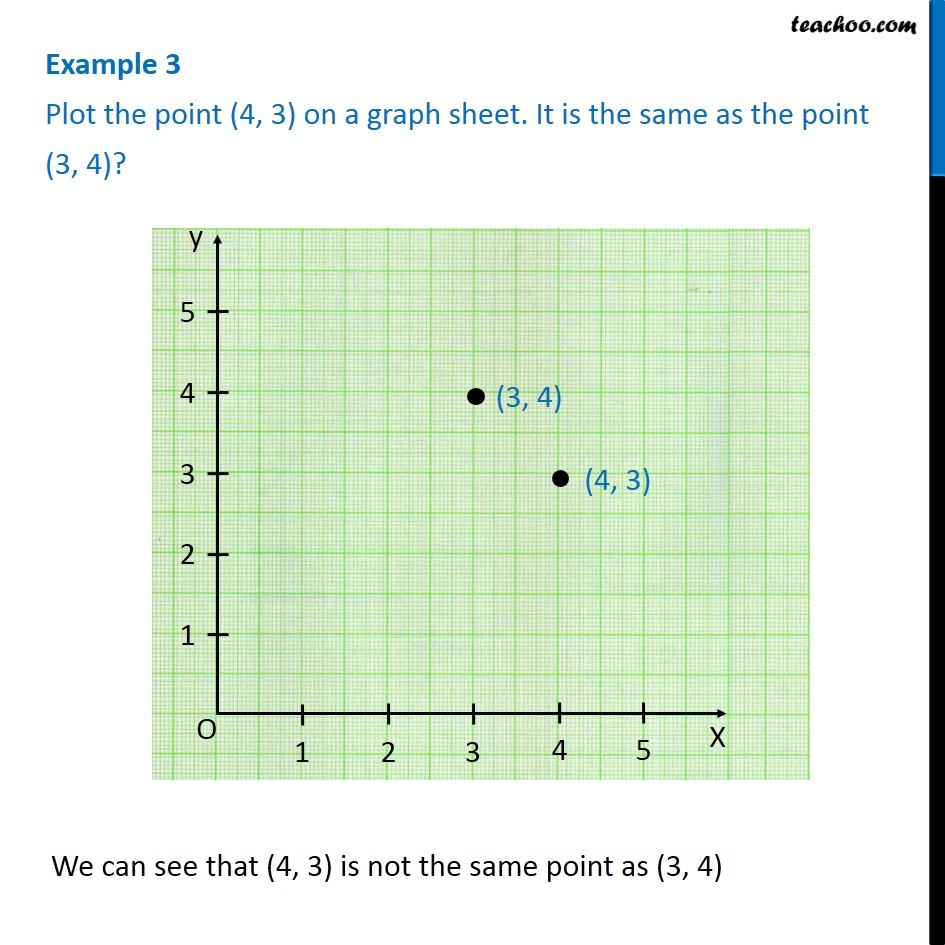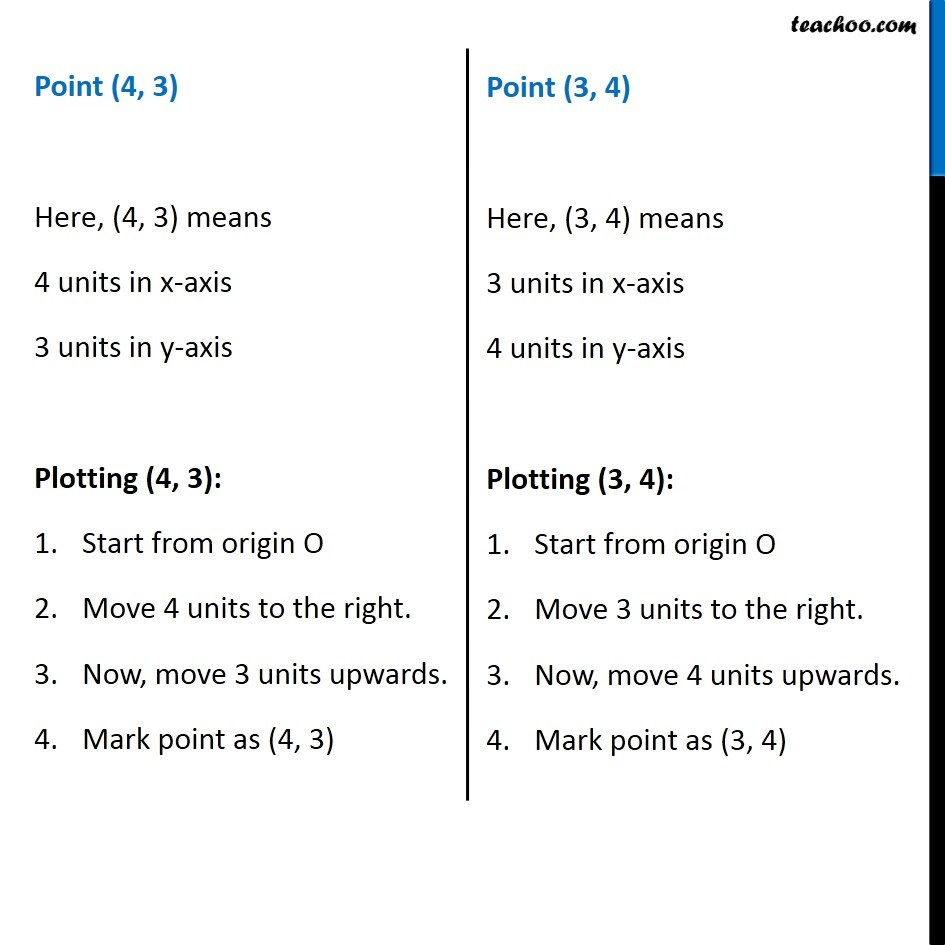1. Chapter 15 Class 8 Introduction to Graphs
2. Concept wise
3. Linear Graphs

Transcript

Example 3 Plot the point (4, 3) on a graph sheet. It is the same as the point (3, 4)? We can see that (4, 3) is not the same point as (3, 4) Point (4, 3) Here, (4, 3) means 4 units in x-axis 3 units in y-axis Plotting (4, 3): Start from origin O Move 4 units to the right. Now, move 3 units upwards. Mark point as (4, 3) Point (3, 4) Here, (3, 4) means 3 units in x-axis 4 units in y-axis Plotting (3, 4): Start from origin O Move 3 units to the right. Now, move 4 units upwards. Mark point as (3, 4)

Linear Graphs